GeeksforGeeks App
Open AppBrowser
Continue

# Python sys Module

The sys module in Python provides various functions and variables that are used to manipulate different parts of the Python runtime environment. It allows operating on the interpreter as it provides access to the variables and functions that interact strongly with the interpreter. Let’s consider the below example.

Example:

## Python3

 `import` `sys`` ` ` ` `print``(sys.version)`

Output:

```3.6.9 (default, Oct  8 2020, 12:12:24)
[GCC 8.4.0]```

In the above example, sys.version is used which returns a string containing the version of Python Interpreter with some additional information. This shows how the sys module interacts with the interpreter. Let us dive into the article to get more information about the sys module.

## Input and Output using sys

The sys modules provide variables for better control over input or output. We can even redirect the input and output to other devices. This can be done using three variables –

• stdin
• stdout
• stderr

stdin: It can be used to get input from the command line directly. It is used for standard input. It internally calls the input() method. It, also, automatically adds ‘\n’ after each sentence.

Example:

## Python3

 `import` `sys `` ` ` ` `for` `line ``in` `sys.stdin: ``    ``if` `'q'` `=``=` `line.rstrip(): ``        ``break``    ``print``(f``'Input : {line}'``) `` ` `print``(``"Exit"``) `

Output: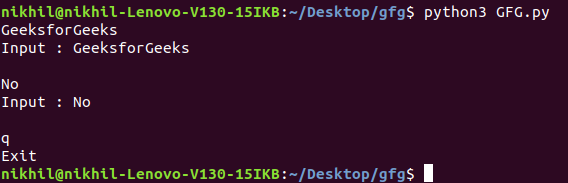stdout: A built-in file object that is analogous to the interpreter’s standard output stream in Python. stdout is used to display output directly to the screen console. Output can be of any form, it can be output from a print statement, an expression statement, and even a prompt direct for input. By default, streams are in text mode. In fact, wherever a print function is called within the code, it is first written to sys.stdout and then finally on to the screen.

Example:

## Python3

 `import` `sys`` ` ` ` `sys.stdout.write(``'Geeks'``)`

Output

`Geeks`

stderr: Whenever an exception occurs in Python it is written to sys.stderr.

Example:

## Python3

 `import` `sys `` ` ` ` `def` `print_to_stderr(``*``a): `` ` `    ``# Here a is the array holding the objects ``    ``# passed as the argument of the function ``    ``print``(``*``a, ``file` `=` `sys.stderr) `` ` `print_to_stderr(``"Hello World"``) `

Output: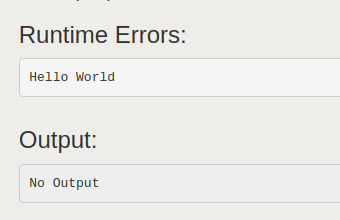## Command Line Arguments

Command-line arguments are those which are passed during the calling of the program along with the calling statement. To achieve this using the sys module, the sys module provides a variable called sys.argv. It’s main purpose are:

• It is a list of command-line arguments.
• len(sys.argv) provides the number of command-line arguments.
• sys.argv is the name of the current Python script.

Example: Consider a program for adding numbers and the numbers are passed along with the calling statement.

## Python3

 `# Python program to demonstrate``# command line arguments`` ` `import` `sys`` ` `# total arguments``n ``=` `len``(sys.argv)``print``(``"Total arguments passed:"``, n)`` ` `# Arguments passed``print``(``"\nName of Python script:"``, sys.argv[``0``])`` ` `print``(``"\nArguments passed:"``, end ``=` `" "``)``for` `i ``in` `range``(``1``, n):``    ``print``(sys.argv[i], end ``=` `" "``)``     ` `# Addition of numbers``Sum` `=` `0`` ` `for` `i ``in` `range``(``1``, n):``    ``Sum` `+``=` `int``(sys.argv[i])``     ` `print``(``"\n\nResult:"``, ``Sum``)`

Output: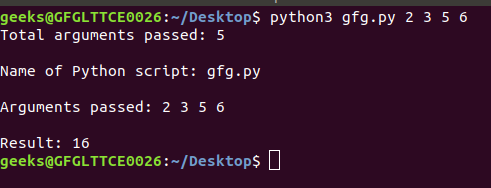## Exiting the Program

sys.exit([arg]) can be used to exit the program. The optional argument arg can be an integer giving the exit or another type of object. If it is an integer, zero is considered “successful termination”.

Note: A string can also be passed to the sys.exit() method.

Example:

## Python3

 `# Python program to demonstrate ``# sys.exit() `` ` ` ` `import` `sys `` ` ` ` `age ``=` `17`` ` ` ` `if` `age < ``18``: ``     ` `    ``# exits the program ``    ``sys.exit(``"Age less than 18"``)     ``else``: ``    ``print``(``"Age is not less than 18"``) `

Output:

```An exception has occurred, use %tb to see the full traceback.

SystemExit: Age less than 18```

## Working with Modules

sys.path is a built-in variable within the sys module that returns the list of directories that the interpreter will search for the required module.

When a module is imported within a Python file, the interpreter first searches for the specified module among its built-in modules. If not found it looks through the list of directories defined by sys.path.

Note: sys.path is an ordinary list and can be manipulated.

Example 1: Listing out all the paths

## Python3

 `import` `sys`` ` ` ` `print``(sys.path)`

Output: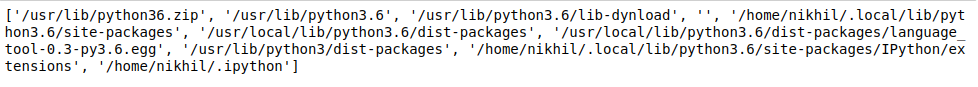Example 2: Truncating the value of sys.path

## Python3

 `import` `sys`` ` `# Removing the values``sys.path ``=` `[]`` ` `# importing pandas after removing``# values``import` `pandas`

Output:

`ModuleNotFoundError: No module named 'pandas'`

sys.modules return the name of the Python modules that the current shell has imported.

Example:

## Python3

 `import` `sys`` ` ` ` `print``(sys.modules)`

Output: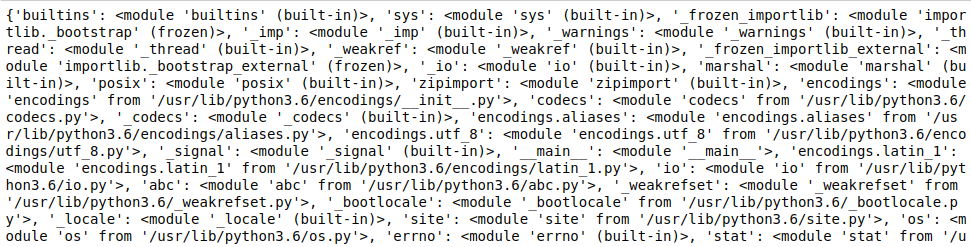## Reference Count

sys.getrefcount() method is used to get the reference count for any given object. This value is used by Python as when this value becomes 0, the memory for that particular value is deleted.

Example:

## Python3

 `import` `sys`` ` `a ``=` `'Geeks'`` ` `print``(sys.getrefcount(a))`

Output

`4`

## More Functions in Python sys

My Personal Notes arrow_drop_up